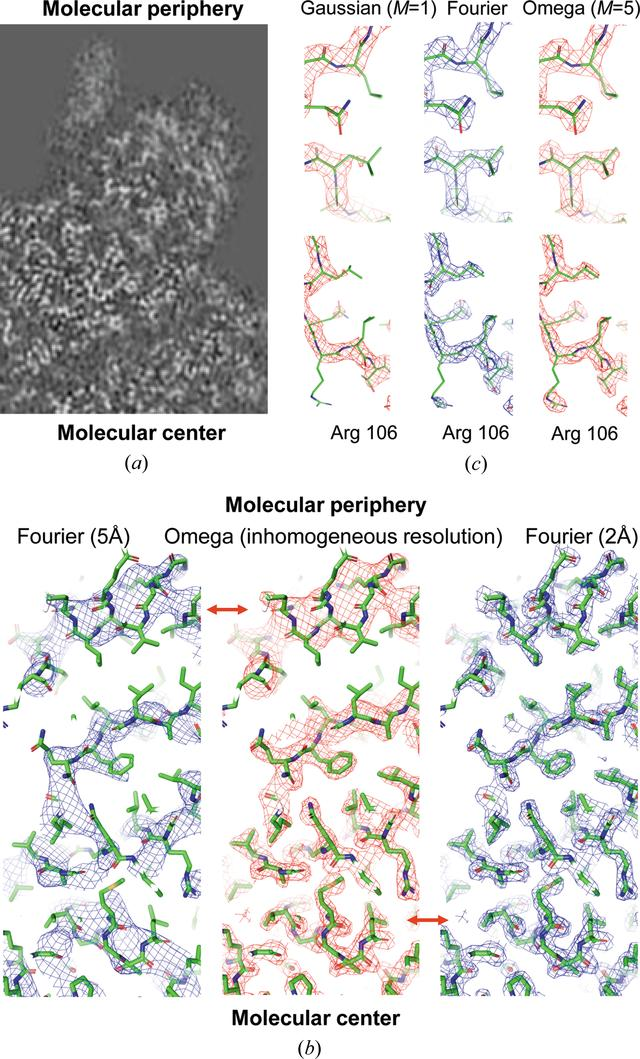disable zoom     view article Figure 1 Maps of inhomogeneous resolution. (a) A fragment of a cryoEM map (von Loeffelholz et al., 2018; EMDB 4261) illustrates a decrease of the local resolution from the molecular center to the periphery. (b) Test model Ω-map (equation 15) of an inhomogeneous resolution varying from 2 Å in the molecular center to 5 Å at the molecular periphery calculated in a single run by the shell decomposition (middle), and the maps calculated by the Fourier procedure with the resolution of 5 Å (left) and 2 Å (right). Red arrows mark the similarity of different parts of the Ω-map with the Fourier maps of different resolution. (c) Fragments of the 2 Å resolution maps contoured to show an equal volume (Urzhumtsev et al., 2014). The map in the middle was calculated by the standard Fourier procedure. The left-hand map calculated as the sum of the Gaussian approximation, M = 1, to the atomic images (no ripples included) reveals the density for some side chains poorly. The right-hand map was calculated by the shell decomposition (equation 15) with M = 5 and reproduces the Fourier map correctly. Poor density for the Arg106 residue in the Ω- and Fourier maps can be attributed to large displacement parameters of its atoms. This figure was prepared with PyMol (DeLano, 2002).

IUCrJ
Volume 9| Part 6| November 2022| Pages 728-734
ISSN: 2052-2525